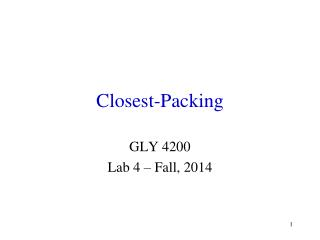DownloadDownload PresentationClosest-Packing

# Closest-Packing

Télécharger la présentation## Closest-Packing

- - - - - - - - - - - - - - - - - - - - - - - - - - - E N D - - - - - - - - - - - - - - - - - - - - - - - - - - -
##### Presentation Transcript

1. Closest-Packing GLY 4200 Lab 4 – Fall, 2014

2. Hexagonal Closest-Packing (HCP) • Third layer is directly over the first layer • Repeat is ABABAB…

3. Cubic Closest-Packing (CCP) • Third layer is distinct from the first two layers • Repeat is ABCABCABC….

4. Intralayer Voids • Space between the spheres, within the layer, is a trigonal planar void • A void is a space which may be occupied by a small ion, usually a cation

5. Void:Sphere Ratio • Ratio of voids/spheres • Around each sphere there are six voids • Around each void there are three spheres • The ratio of voids/spheres is therefore 2:1

6. Interlayer Voids • Both types of packing have empty places (voids) between layers • There are two types of voids • Each has a different CN

7. Assignment • Find out what types of voids are present • Find the ratio of voids/sphere for each type • Find the ratio of the voids to one another